# Electric current resistance and resistivity Problems

Question 1:
A cylindrical tube of length L has inner radius a and outer radius b as shown below in the figure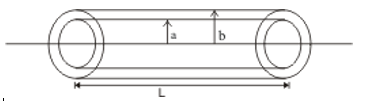Given that ρ is the resistivity of the material. Find the resistance of the tube between its ends.

Question 2
α1 and α2  are the temperature coefficient  of the two resistors R1 and R2 at any  temperature T0 0 C. Find the equivalent temperature coefficient  of their equivalent resistance  if both R1 and R2 are connected in series combination. Assume that  α1 and α2  remain same with change in temperature

Question 3
If a copper wire is stretched to make it .2%  longer. Find the percentage change in the resistance

Question 4:
An electric current of 5 A passes through a circuit containing three wires(A,B,C) of the same material .Length of the wires in the circuit are in the ratio 2:3:4 And their diameters are in the ratio 3:4:5.Find the amount of current flowing in each branch  of the circuit  when wires are arranged in parallel combination

Question 5:
Find the equivalent resistance between point A and B in following combination of resistors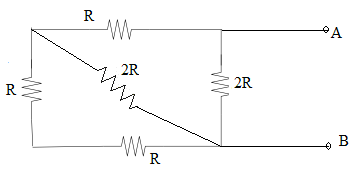Question 6:
The current density across a cylindrical conductor of Radius A varies according to the following relation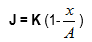Where K is the constant vector across the length of the conductor and x is the distance from axis.
Find the current through cylindrical cross-section

Question 7
Consider the figure given below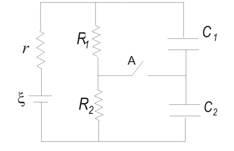Where E=10V is a source of emf with internal resistance r=0.5Ω which is connected to two resistors R1=2Ω and R2=4Ω. Two capacitors C1=0.02µF and C2=0.05µF are connected in parallel to the resistors. Calculate
(a)Current in the circuit and charges Q1 and Q­2 on the capacitors when steady state is reached with switch A open.
(b)Current in the circuit and charges Q1 and Q­2 on the capacitors when steady state is reached with switch A closed

Question 8
Consider the infinite network of resistors as shown below in the figure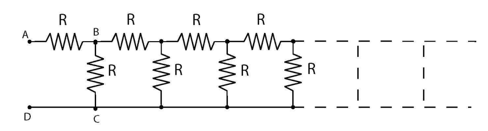Each resistor in the infinite network has a value of 2Ω. Find the current drawn from 12V supply of internal resistance 0.5Ω.